# Exercises: Chapter 3, Section 5

1. Suppose that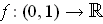is a non-negative continuous function. Show that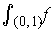exists if and only if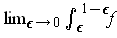exists.

For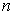a natural number, define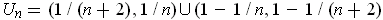and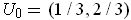. Consider a partition of unity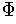subordinate to the cover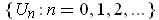. By summing the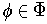with the same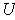in condition (4) of Theorem 3-11, one can assume that there is only one function for each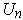, let it be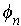. Nowexists if and only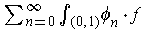converges. But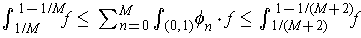. So the sum converges if and only ifexists.

2. Let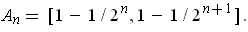Suppose thatsatisfies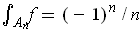and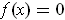for all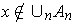. Show thatdoes not exist, but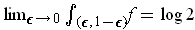.

Take a partition of unitysubordinate to the cover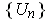where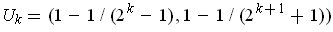for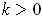. As in part (a), we can assume there is only one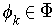as in condition (4) of Theorem 3-11. Consider the convergence of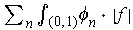. One has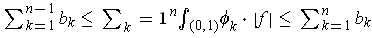where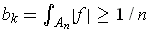. It follows that the sum in the middle does not converge as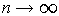and sodoes not exist.

The assertion that. If not necessrily true. From the hypothesis, we only know the values of the integral of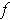on the sets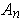, but don't know howbehaves on other intervals -- so it could be that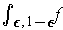may not even exist for all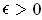To correct the situation, let us assume thatis of constant sign and bounded on each set. Thenis bounded on each interval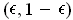and so by Theorem 3-12, the integral in the extended sense is same as the that in the old sense. Clearly, the integral in the old sense is monotone on each interval of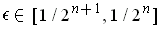, and the limit is just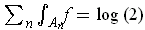.

1. Letbe a closed set contained in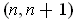. Suppose that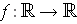satisfiesand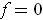outside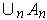. Find two partitions of unityand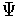such that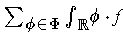and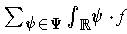converge absolutely to different values.

The sums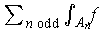and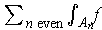have terms of the same sign and are each divergent. So, by re-ordering the terms of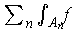, one can make the sum approach any number we want; further this can be done so that there are sequences of partial sums which converge monotonically to the limit value. By forming open covers each set of which consists of intervalsfor the sum of terms added to each of these partial sums, one gets covers of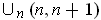. Becauseis zero outside, one can `fatten' up the covering sets so that they are a cover of the real numbers no smaller than 1 without adding any points whereis non-zero. Finally, one can take a partition of unity subordinate to this cover. By using arrangements with different limiting values, one gets the result.